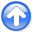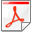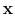Basic operators and adjointsNext: Backsolving, polynomial division and Up: FAMILIAR OPERATORS Previous: Spray and sum :

Causal and leaky integration

Causal integration is defined as:(17)

Leaky integration is defined as:(18)

As, leaky integration becomes causal integration. The word leaky'' comes from electrical circuit theory in which the voltage on a capacitor would be the integral of the current if the capacitor did not leak electrons.

Sampling the time axis gives a matrix equation that we should call causal summation,'' but we often call it causal integration.'' Equation (19) represents causal integration forand leaky integration for.(19)

(The discrete world is related to the continuous byand in some applications, the diagonal is 1/2 instead of 1.) Causal integration is the simplest prototype of a recursive operator. The coding is trickier than that for the operators we considered earlier. Notice when you computethat it is the sum of 6 terms, but that this sum is more quickly computed as. Thus, equation (19) is more efficiently thought of as the recursion(20)

(which may also be regarded as a numerical representation of the differential equation.)

When it comes time to think about the adjoint, however, it is easier to think of equation (19) than of equation (20). Let the matrix of equation (19) be called. Transposing to getand applying it togives us something back in the space of, namely. From it we see that the adjoint calculation, if done recursively, needs to be done backward, as in:(21)

Thus, the adjoint of causal integration is anticausal integration.

A module to do these jobs is leakint. The code for anticausal integration is not obvious from the code for integration and the adjoint coding tricks we learned earlier. To understand the adjoint, you need to inspect the detailed form of the expressionand take care to get the ends correct. Figure 5 illustrates the program for.

api/c/causint.c
 t = 0.; if (adj) { for (i=nx-1; i >= 0; i-) { t += yy[i]; xx[i] += t; } } else { for (i=0; i <= nx-1; i++) { t += xx[i]; yy[i] += t; } }

causint
Figure 5.
in1 is an input pulse. C in1 is its causal integral. C' in1 is the anticausal integral of the pulse. A separated doublet is in2. Its causal integration is a box, and its anticausal integration is a negative box. CC in2 is the double causal integral of in2. How can an equilateral triangle be built?Later, we consider equations to march wavefields up toward the Earth surface, a layer at a time, an operator for each layer. Then, the adjoint starts from the Earth surface and marchs down, a layer at a time, into the Earth.Basic operators and adjointsNext: Backsolving, polynomial division and Up: FAMILIAR OPERATORS Previous: Spray and sum :

2014-09-27